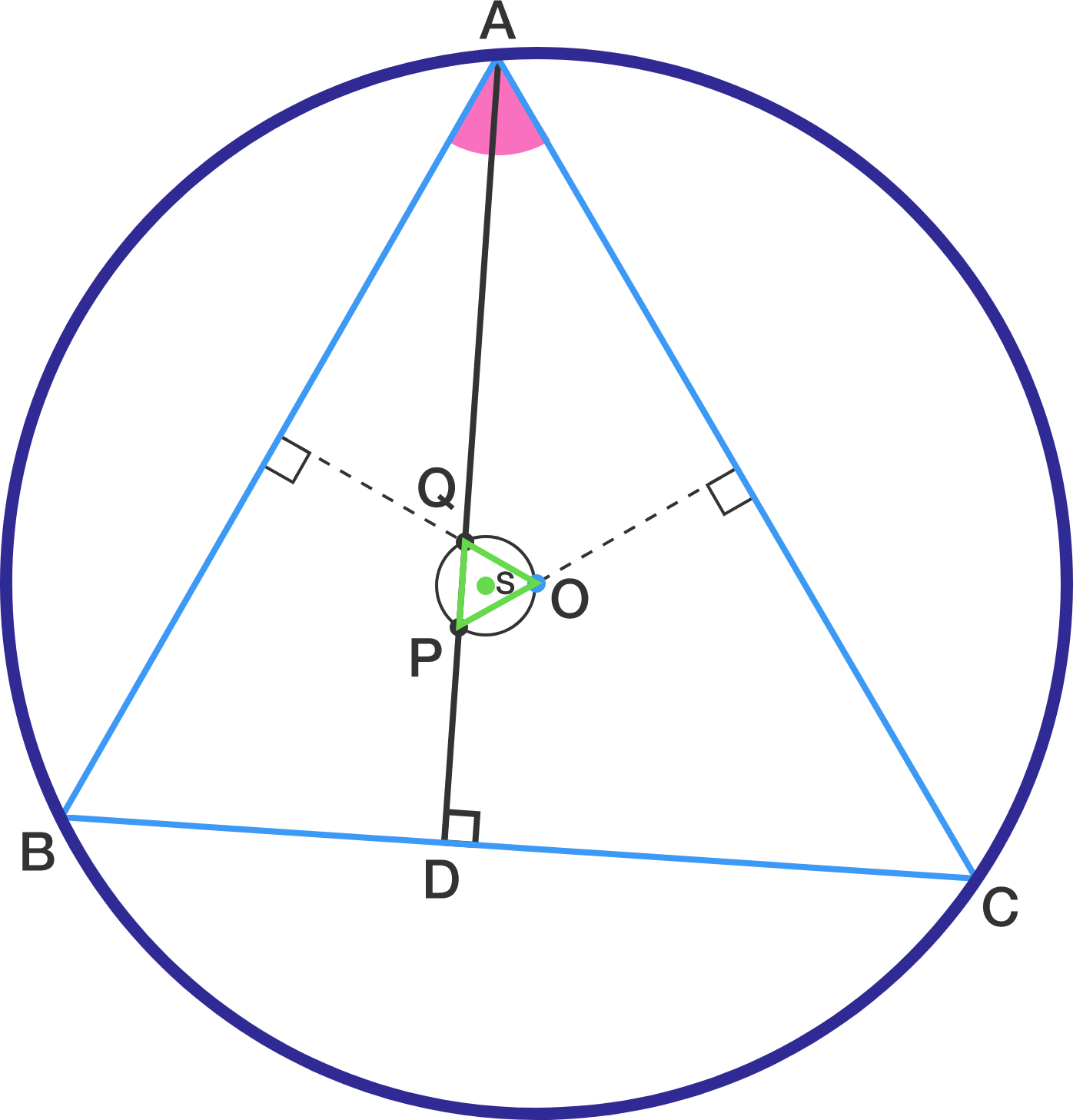# What Is This Point?

Geometry Level 5Let $\triangle ABC$ be an acute angled triangle with side lengths $AB= 5, BC= 7, CA= 8.$ Let $D$ be the foot of perpendicular from $A$ to $BC,$ and let $O$ be its circumcenter. The feet of perpendiculars from $O$ to $AB$ and $AC$ intersect $AD$ at points $Q$ and $P$ respectively. Let $S$ be the circumcenter of $\triangle OPQ.$ If $\cos (\angle CAS) = \frac{ a \sqrt{b} } { c}$ for some square free integer $b$ and coprime positive integers $a$ and $b$, then find $a+b+c$.

Details and assumptions
- This problem is adapted from an ARO 10th grade geometry problem.

×